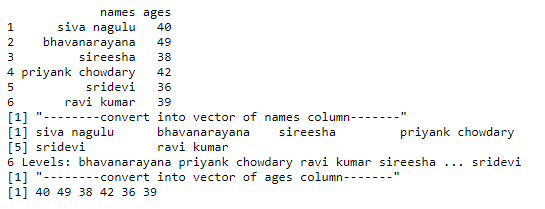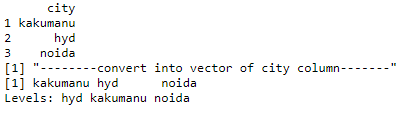# How to extract column from data frame as vector in R ?

• Last Updated : 07 Apr, 2021

In this article, we are going to convert dataframe column into a vector in R Programming Language.

### Steps –

• Create vectors
• Create a dataframe by passing these vectors
• Convert dataframe column using“[[]]” operator (indexing). [[]] is used to access the dataframe column.It is used to index the dataframe.

Syntax:

dataframe_name[[‘column_name’]]

• Display vectors

Example 1:

## R

 `# creating a vector for names``names=``c``(``'siva nagulu'``,``'bhavanarayana'``,``'sireesha'``,``        ``'priyank chowdary'``,``'sridevi'``,``'ravi kumar'``)`` ` `# creating a vector for ages``ages=``c``(40,49,38,42,36,39)`` ` `#create a dataframe using these vectors``dataframe=``data.frame``(names,ages)``print``(dataframe)`` ` `# converting ages dataframe column into vector``# by passing as index``my_data = dataframe[[``'names'``]]``print``(``"--------convert into vector of names column-------"``)``print``(my_data)`` ` `my_data = dataframe[[``'ages'``]]``print``(``"--------convert into vector of ages column-------"``)``print``(my_data)`

Output:Example 2:

## R

 `# creating a vector for city``city=``c``(``'kakumanu'``,``'hyd'``,``'noida'``)`` ` `# create a dataframe using this vector``dataframe=``data.frame``(city)``print``(dataframe)`` ` `# converting city dataframe column into vector``# by passing as index``my_data = dataframe[[``'city'``]]``print``(``"--------convert into vector of city column-------"``)``print``(my_data)`

Output:My Personal Notes arrow_drop_up